# Blocked Flow - Gas Relief - Intergraph Smart Instrumentation - 13.1 - Reference - Hexagon PPM

## Integraph Smart Instrumentation Sizing Equations

Language
English (United States)
Product
Intergraph Smart Instrumentation
Search by Category
Reference
Smart Instrumentation Version
13.1
1. Calculate upstream relieving pressure:

*Pa [psi-a]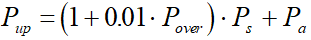2. Calculate total back pressure: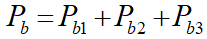3. Calculate critical flow throat pressure: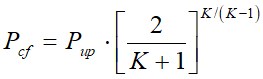4. Effective coefficient of discharge: Kd = 0.975.

5. The condition Pb < P(Pb [psi-a]) determines:

Case A - Critical flow.

1. Calculate coefficient for critical flow equation: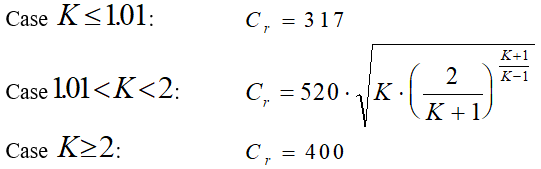2. Calculate capacity correction factor due to back pressure (if Kb is not entered by user).

For conventional and Pilot operated valves, Kb = 1.

For Bellows valve:

Y= Pb / Ps

1.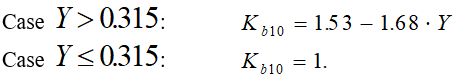2.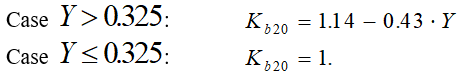3.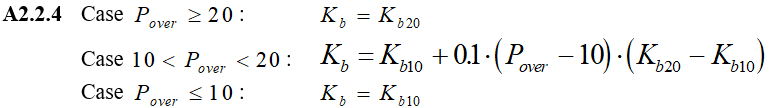3. Estimate required effective discharge area: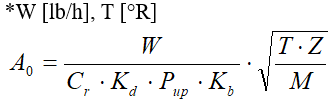Case B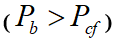- Sub-critical flow: Bellow valve

1. Calculate Cr: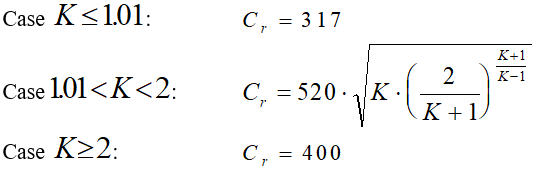2. If capacity correction factor due to back pressure is not entered by user Kb =1.

3. Estimate required effective discharge area: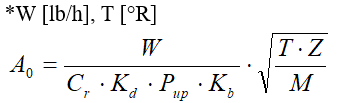Case C- Sub-critical flow - Conventional and Pilot operated valves

1. Calculate coefficient for sub-critical flow equation: *Pb [psi-a].

1.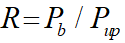2.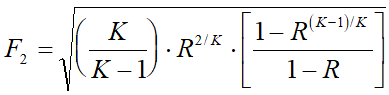2. Estimate required effective discharge area: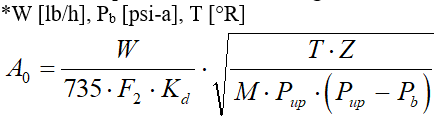6. For Rupture disk application, calculate required effective discharge area:

A = A0 /Kcc